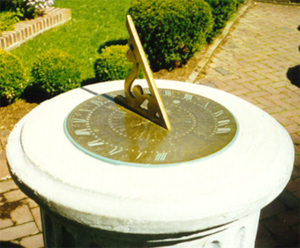The sundial consists of the gnomon and the dial plate. For a horizontal sundial (like you find in your garden), the gnomon rises at an angle equal to the sundial site's latitude.

But what about the hour lines on the dial plate? They must also match the sundial's latitude. Bannekar's sundial showed how to do this graphically. Here we'll use a simple formula and allow our screen calcuator to do the work (See column on the right)

The formula for calculating the hour lines (theta) on a horizontal sundial is:

tan(theta) = tan(HA) x sin(lat)

where:

theta = the resulting dial hour angle measured from the noon line (- is left of the noon line, + is right of the noon line)

HA = the hour angle of the sun from the noon meridian, expressed in (+/-) degrees. The hours are minus in the morning (ante meridian) and positive in the afternoon (post meridian).

lat = sundial site latitude, in degrees

We need to determine the dial angle theta for each hour angle HA of the sun. We measure HA using the meridian as the reference. At 10 AM, the sun is 2 hours before the meridian. At 3:15 PM the sun is 3 hours 15 min ( or 3 ¼ hour) after the meridian.

Since the earth rotates 360 degrees in 24 hours, that's 15 degrees in 1 hour. So 10 AM can be expressed as the hour angle of -2 x 15o = -30o (Notice the minus sign) At 3:15 PM, the hour angle is 3.25 x 15o = 48.75o (Notice this is positive)